Properties
Translations
Rotations
Reflections
Identifying Rules
Recognize the transformation
100

The word for preserving same size and same shape?

What is congruency?

100

The word that describes translating.

Slide

100

The word that describes rotating

turning

100

This word is another way of saying reflecting

Flip

100

This rule describe translating up 6 units.

(x, y+6)

100

The graph depicts a rigid transformation. What is the rule? Give the words and the notation.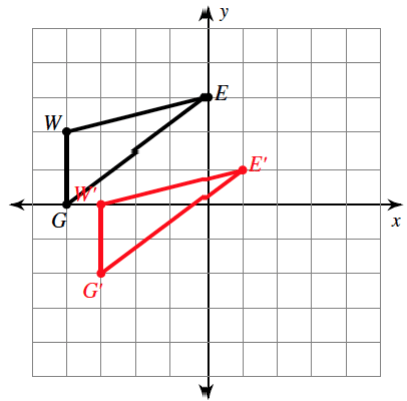1 unit right 2 units down: (x + 1, y - 2)

200

Which transformations doesn't change orientation?

translations

200

Adding to the x-coordinate moves a figure in this direction.

to the right

200

If both coordinates change signs what transformation occurred?

180 degree rotation

200

This coordinate stays the same when a figure is reflected over the y-axis.

the y-coordinate.

200

Describe this transformation rule: (x, + or - y)

reflect over the x-axis?

200

The given graph depicts a rigid transformation.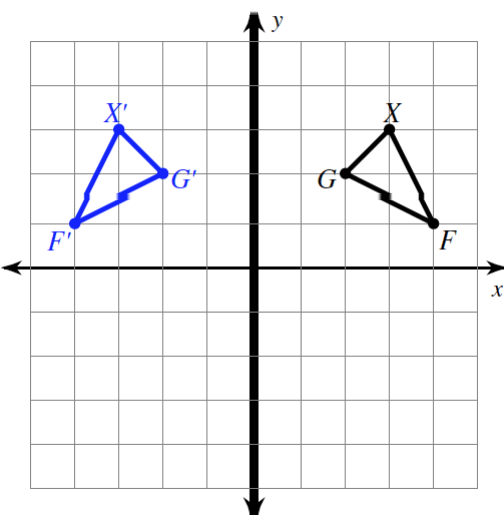What is a reflection in the y-axis?

300

The picture below shows this type of transformation.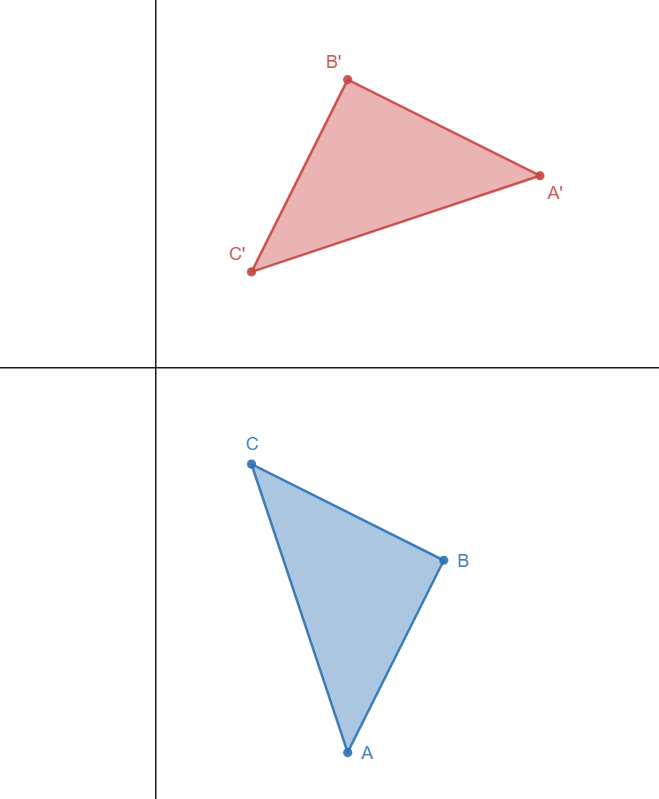Rotation

300

If (1, 6) is translated to the left 3 and down 1, what are the new coordinates?

(-2, 5)

300

The segment below has been rotated 90o in what direction?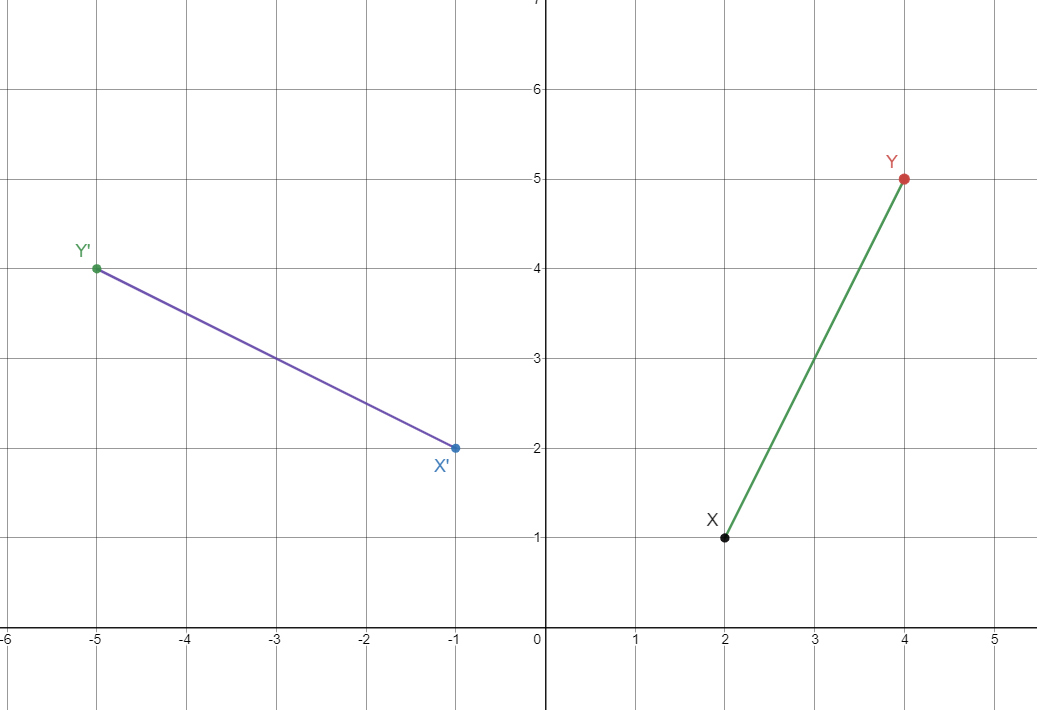counter clockwise

300

This coordinate changes when a figure is reflected over the x-axis.

the y-coordinate

300

If (1,5) becomes (3,0) what is the transformation rule?

(x + 2, y - 5)

300

The given graph depict multiple transformations of the pre-image *. This image depicts a Reflection of * across the y-axis.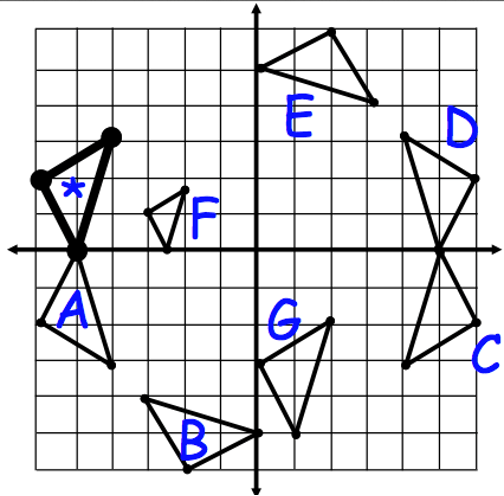What is D?

400

This notation (symbol) is how we indicate the figure from the image.

prime mark A'

400

This is what happens to the coordinates if a figure translates up and to the left.

subtract from the x-coordinate and add to the y-coordinate?

400

This is the coordinate M' after the figure below is rotated 180o clockwise.(-6, -6)?

400

The figure has been reflected over this line.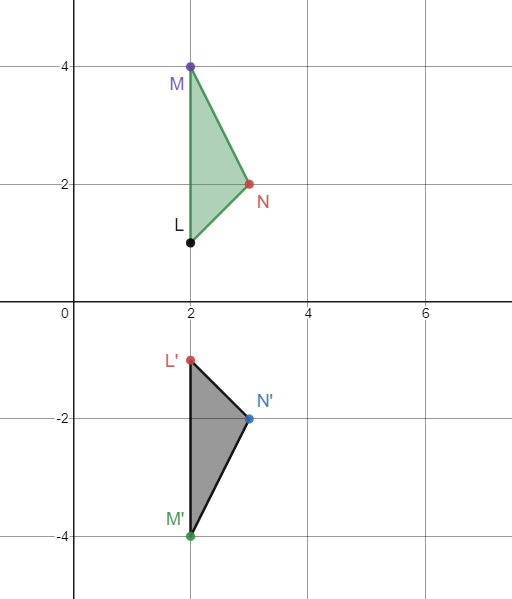the x-axis

400

This rule describes rotating 270o clockwise.

(change the sign of y, x) or 90 degrees CCW

400

The given graph depicts a rigid transformation.What is a 90-degree clockwise rotaition?

500

Which figure is the image in the following reflection: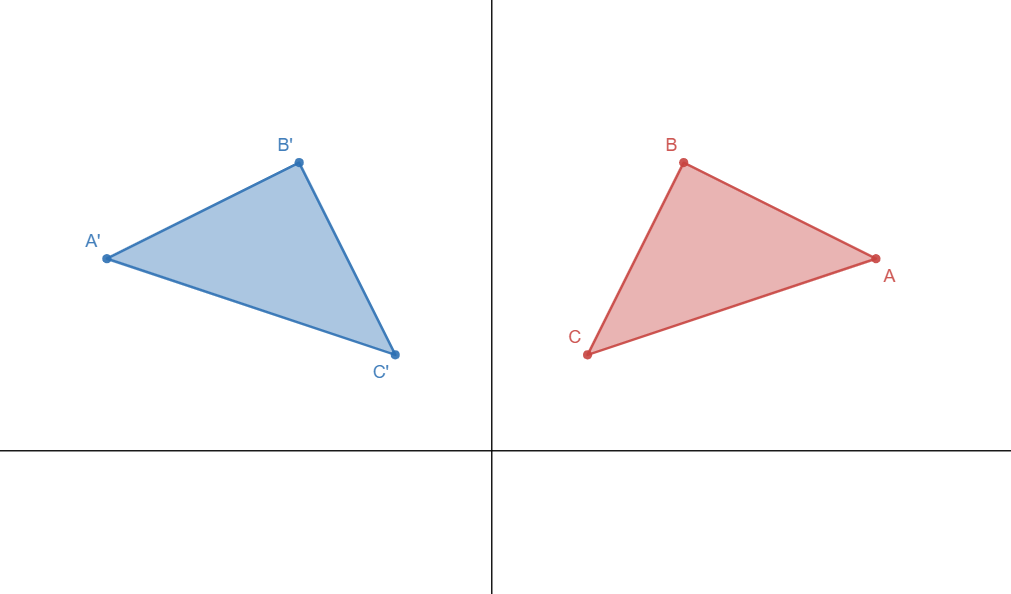the blue triangle

500

In words, what is the translation rule for the figure to image below?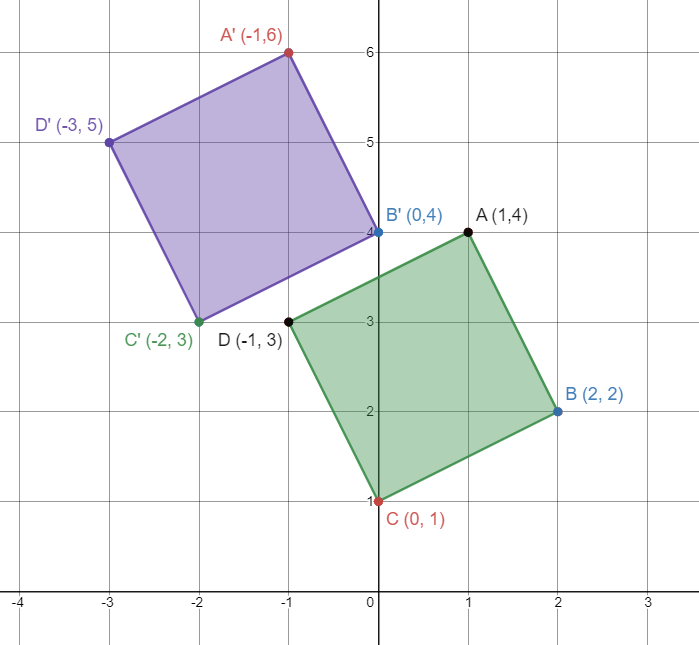left two units, up two units

500

This clockwise rotation is the same as rotating 90o counterclockwise.

270o clockwise

500

Reflect the triangle ABC over Y-axis with side lengths AB =3 units, BC = 4 units and AC = 5 Units. After reflection What is the length of BC?

4 Units.

500

(y, -x) is a description of rotating 270o counterclockwise or rotating 90o clockwise. True or False

True

500

The given graph shows rigid transformations of the pre-image . Triangle *  will have a  Rotations of  90-degrees counterclockwise about the origin. Where  will the new figure be?What is figure B?

600

Name the rigid transformations.

translation, reflection, rotations

600

This is the algebraic rule of a translation that moves a figure 2 units left and 3 units up.

(x, y) → (x − 2, y + 3)

600

What is the rotation from pre image to image?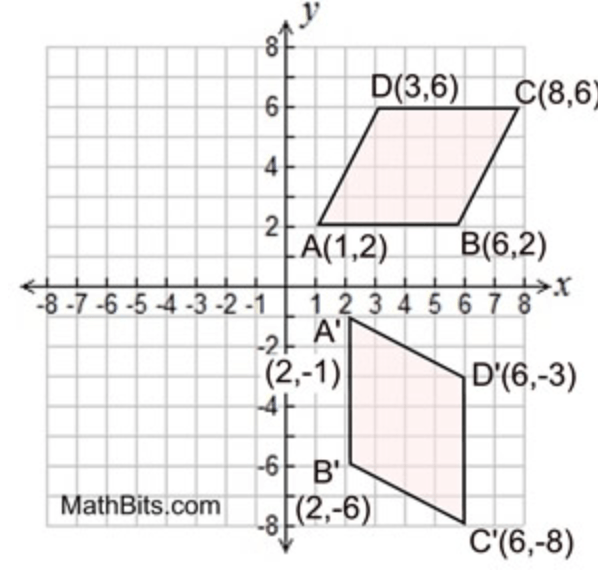90 degrees clockwise or 270 degree counter clockwise.

600

Reflect triangle PQR over X axis, what is the angle measure for P' Q' R'? Angle Measure forP = 60, R=90, Q = 30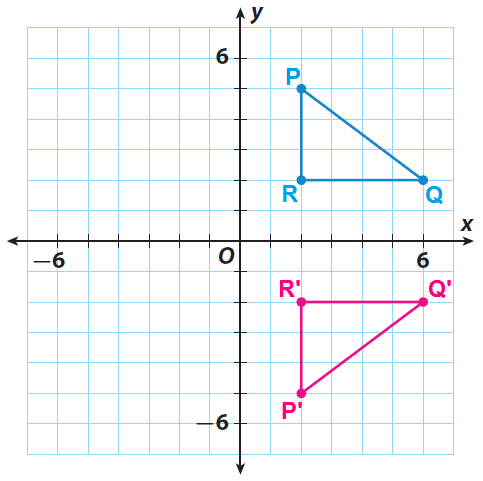R' 90, P' 60, Q' 30

600

This is the rule for the following transformationrotation 90 degree counterclockwise about the origin

(𝑥, 𝑦) → (−𝑦,𝑥)

600

The given graph represents transformations of the pre-image *. This figure shows a Rotation of the pre-image clockwise about the origin.What is figure C?

700

What is congruency? How do you prove congurency?

Two figures are congruent if one can be mapped onto another through series of rigid transformations.

700

Give the verbal description for (x,y) -> (x-4,y+0)

the figure moves 4 units to the left.

700

What would be the transformation in the illustration?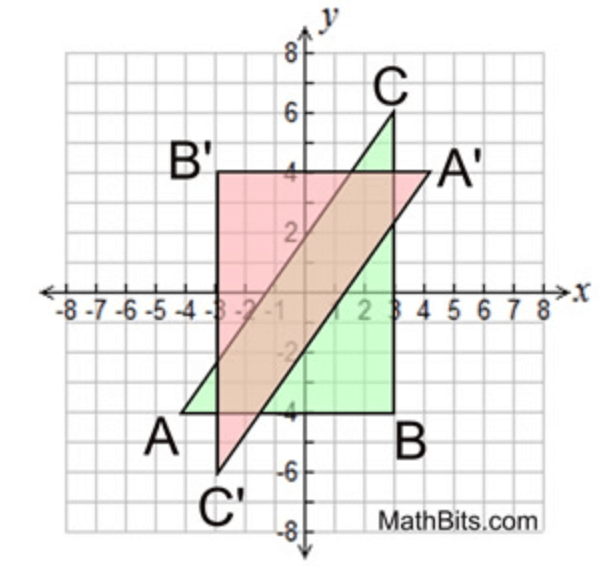180 degree rotation

700

A y-axis representation of the word MOON would produce this word. An x-axis representation of the word WOW would produce this word.

NOOM and MOM?

700

What is the rule for the given transformation.Reflection across the y-axis (𝑥, 𝑦) → (−𝑥,𝑦)

700

These two transformations have occurred to the given figure.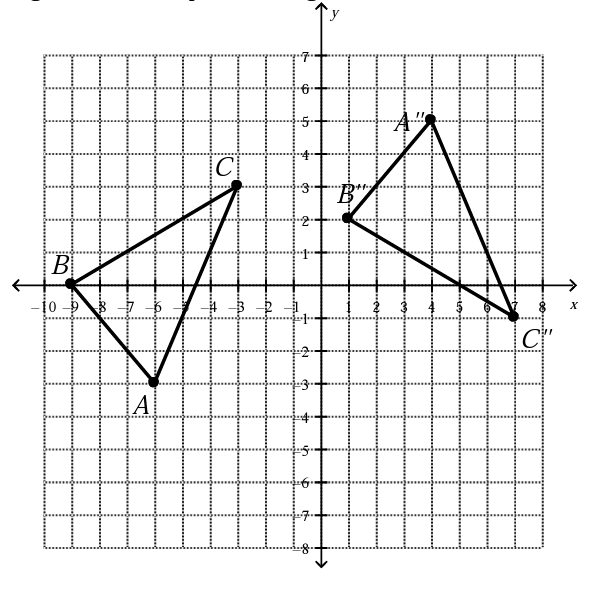What is a Reflection across the x-axis and then translated 10 units right and 2 units up.

Click to zoom